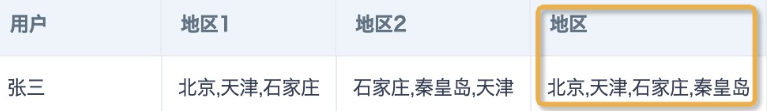# Spark SQL数组处理函数及应用

## 定义

数组（Array）是有序的元素序列，组成数组的各个变量称为数组的元素。数组是在程序设计中，为了处理方便把具有相同类型的若干元素按有序的形式组织起来的一种形式。按数组元素的类型不同，数组又可分为数值数组、字符数组等各种类别。数组在各个编程语言里处理方式不同，本文仅列出数组在Spark SQL里的函数以及应用案例。

## 特点

1. 数组是相同数据类型的元素的集合。

2. 数组中的各元素的存储是有先后顺序的，它们在内存中按照这个先后顺序连续存放在一起。

3. 数组元素用整个数组的名字和它自己在数组中的顺序位置（索引）来查询。

4. 数组在数据库里展示为整体用方括号括起来的类字符串，每个元素用逗号隔开。例如 [1, 2, 3]。

## 主要函数

#### 数组生成与转换

 用途 函数 举例 结果 多行合并为一行，返回不去重的数组 collect_list(expr) collect_list([字段])                                  [字段]值分别为 A,A,B,B,C,D [A,A,B,B,C,D] 多行合并为一行，返回去重后的数组 collect_set(expr) collect_set([字段])                                     [字段]值分别为 A,A,B,B,C,D [A,B,C,D] 多列合并为一列 array(expr, ...) array(1, 2, 3) [1,2,3] 用分隔符拆分字符串，返回数组。 split(str, regex[, limit])                     [, limit]限制拆分元素数，可省略 split('A-B-C', '-')；                             split('A-B-C', '-',2) [A,B,C];                                     [A,B-C] 一行拆分为多行 explode(expr) explode(array('A','B')) A                                                B 把数组里元素用分隔符拼接为字符串 array_join(array, sep[, nullRep])                             [, nullRep]用来指定null值的替换值，省略时移除null array_join(array('hello', null ,'world'), ' ', ',') hello, world concat_ws(sep[, str | array(str)]+)           null移除，可拼接多个数组和字符串 concat_ws(',',array('hello', null ,'world')) hello,world 把文本按照标点和空格拆分为嵌套数组 sentences(str[, lang, country]) sentences('Hi there! Good morning.') [["Hi","there"],["Good","morning"]] 生成按固定步长递增的序列数组，用于数值和日期时间类型 sequence(start, stop, step) sequence(1, 5)；             sequence(to_date('2021-01-01'), to_date('2021-03-01'), interval 1 month) [1,2,3,4,5];                          [2021-01-01,2021-02-01,2021-03-01]

1. 数据集多行数据合并成一行，一行拆分为多行。主要使用 `concat_ws(collect_set( )),  explode(split( )) `等函数组合使用。具体使用案例请参考：通过ETL处理数据行的合并和拆分

2. 因为数据缺失（例如没有销售记录）导致同环比数据对不上，需要补齐数据，或者其他需要笛卡尔积的场景。例如，使用组合函数 `explode(sequence([起始日期],[结束日期],interval 1 month)) `来补齐日历。具体使用案例请参考：ETL补齐数据方法

3. 去重计数。count(distinct( )) 函数不支持窗口函数用法，数据量不大时可以用 `size(collect_set([字段])over(partition by [分组列名])) `来进行去重计数。具体使用案例请参考：去重计数函数实现开窗

## 数组基础操作

### 常用函数

 用途 函数 举例 结果 对数组元素进行去重 array_distinct(array) array_distinct(array(1, 2, 3, null, 3)) [1,2,3,null] 返回数组元素个数 size(expr) size(array('b', 'd', 'c', 'a')) 4 移除数组里所有的某指定元素 array_remove(array, element)    (null不能移除) array_remove(array(1, 2, 3, null, 3), 3) [1,2,null] 返回某元素重复指定次数组成的数组 array_repeat(element, count) array_repeat('123', 2) ["123","123"] 从固定位置截取固定长度的子数组 slice(x, start, length) slice(array(1, 2, 3, 4), 2, 2) [2,3] 把数组里的所有元素都按照指定方法处理，返回新的数组 transform(expr, func) transform(array(1, 2, 3), x -> x + 1);         transform(array('A','B','C'),(x,i)->i||x) [2,3,4];                                          [0A,1B,2C] 将数组翻转排序 reverse(array) reverse(array(2, 1, 4, 3)) [3,4,1,2] 对数组随机排序 shuffle(array) shuffle(array(1, 20, 3, 5)) [3,1,5,20] 数组排序 array_sort(expr, func)              func指定排序方式，省略时升序, null排最后 array_sort(array('b', 'd', null, 'c', 'a')) ["a","b","c","d",null] sort_array(array[, true/false])    升序(true)时null排前面，降序(false)时null排最后 sort_array(array('b', 'd', null, 'c', 'a'), true) [null,"a","b","c","d"]

## 数组查询与计算

#### 常用函数

 用途 函数 举例 结果 返回数组里最大值 array_max(array) array_max(array(1, 20, null, 3)) 20 返回数组里最小值 array_min(array) array_max(array(1, 20, null, 4)) 1 查询数组里是否存在某元素，返回true/false array_contains(array, value) array_contains(array(1, 2, 3), 2) true 查询元素在数组里的位置/索引 array_position(array, element) array_position(array('A','B','C'), B) 2 查询数组里第n个元素 element_at(array, n)                              n从1开始，为负数时，从后往前查询 element_at(array('A','B','C'), -1) C array[n]        n从0开始，不能为负 array('A','B','C') A 用指定条件/方法筛选数组，返回包含符合条件元素的数组 filter(expr, func) filter(array(1, 2, 3), x -> x % 2 == 1) [1,3] 查询数组里是否有任何元素符合判断条件，返回true/false exists(expr, pred)  需提前过滤null值，否则会导致结果返回null。 exists(array(1, 2, 3), x -> x % 2 == 0) true 查询数组里是否所有元素都符合判断条件，返回true/false forall(expr, pred)  需提前过滤null值，否则会导致结果返回null。 forall(array(1, 2, 3), x -> x % 2 == 0) false 把数组内元素按照二元运算聚合为一个结果值 aggregate(expr, start, merge, finish) aggregate(array(1, 2, 3), 0, (acc, x) -> acc + x)   数组内元素求和 6

#### 常用场景

1. 用户属性为多值，设置数据集行列权限。

1. 多个关键词，互相为“或”的关系，模糊匹配长字符串。

## 多数组处理

#### 常用函数

 用途 函数 举例 结果 拼接数组，元素不去重 expr1 || expr2 array(1, 2, 3) || array(4, 5) || array(6) [1,2,3,4,5,6] concat(col1, col2, ..., colN) concat(array(1, 2, 3), array(4, 5), array(6)) 返回存在于array1，但不存在于array2里的元素列表，元素去重 array_except(array1, array2) array_except(array(1, 2, 3), array(1, 3, 5))  返回array1和array2 交集，结果去重 array_intersect(array1, array2) array_intersect(array(1, 2, 3), array(1, 3, 5)) [1,3] 返回array1和array3 并集，结果去重 array_union(array1, array2) array_union(array(1, 2, 3), array(1, 3, 5)) [1,2,3,5] 查询array1和array2 是否存在非null值的交集，返回true/false arrays_overlap(array1, array2) arrays_overlap(array(1, 2, 3), array(3, 4, 5)) true 把二维数组合并为一维数组 flatten(arrayOfArrays) flatten(array(array(1, 2), array(3, 4))) [1,2,3,4]

#### 常用场景

1. 从长字符串里截取内容。

1. 合并多个长字符串，并且移除字符串中重复的内容。例如，要实现以下效果，可以参考以下组合函数。

```array_join(array_union(split([地区1],','),split([地区2],',')),',')
--或者--
concat_ws(',',array_distinct(split(concat_ws(',',[地区1],[地区2]),',')))```1. 数据集字段格式为单层或者多层嵌套json数组, 需要提取json内容。相关案例：如何使用ETL解析json

12 人点赞过